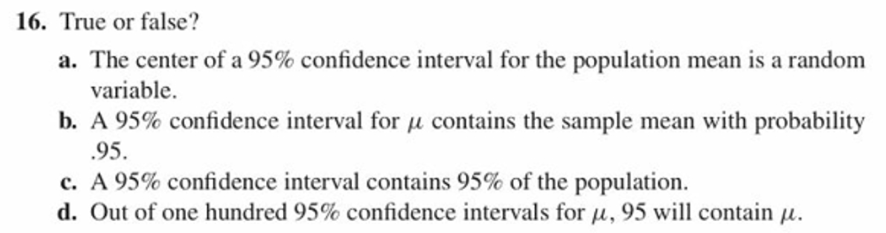# 16. True or false?a. The center of a 95% confidence interval for the population mean is a randomvariable.b, A 95% confidence interval for ,1 contains the sample mean with probability95C. A 95% confidence interval contains 95% of the population.d. Out of one hundred 95% confidence intervals for 14. 95 will contain 14.

Question
14 viewshelp_outlineImage Transcriptionclose16. True or false? a. The center of a 95% confidence interval for the population mean is a random variable. b, A 95% confidence interval for ,1 contains the sample mean with probability 95 C. A 95% confidence interval contains 95% of the population. d. Out of one hundred 95% confidence intervals for 14. 95 will contain 14. fullscreen
check_circle

Step 1

Hello there ! I see there are more than three subparts in the question. According to our policies can answer only three subparts. Do find the solutions for a,b,c. If you have doubt in remaining parts, kindly request as a new question.

Step 2

Given "The center of a 95% confidence interval for population mean is a randon variable."

The formula of confidence interval is given below

Here we can see that the boundaries of confidence interval is (sample mean - margin of error and sample mean + margin of error). So the center of the confidence interval is nothing but (Xbar) Sample mean.  The sample mean will vary from sample to sample and so as the sample standard deviation. So we can say that the center of confidence interval for population mean (Xbar) is random. So the given statement is TRUE.

Step 3

Given " A 95% confidence interval for µ contains sample mean with probability 0.95."

The confidence interval for µ is centered with sample mean. Which we say as the best estimate of the population me...

### Want to see the full answer?

See Solution

#### Want to see this answer and more?

Solutions are written by subject experts who are available 24/7. Questions are typically answered within 1 hour.*

See Solution
*Response times may vary by subject and question.
Tagged in

### Measures